# 5) dợ = E•dA, where E = (28 V/m?) xy î - (8.3 V/m) sin(2z/n) k,...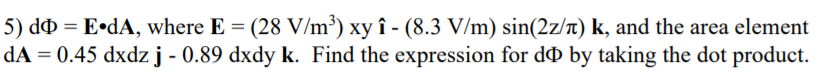5) dợ = E•dA, where E = (28 V/m?) xy î - (8.3 V/m) sin(2z/n) k, and the area element dA = 0.45 dxdz j - 0.89 dxdy k. Find the expression for dỏ by taking the dot product.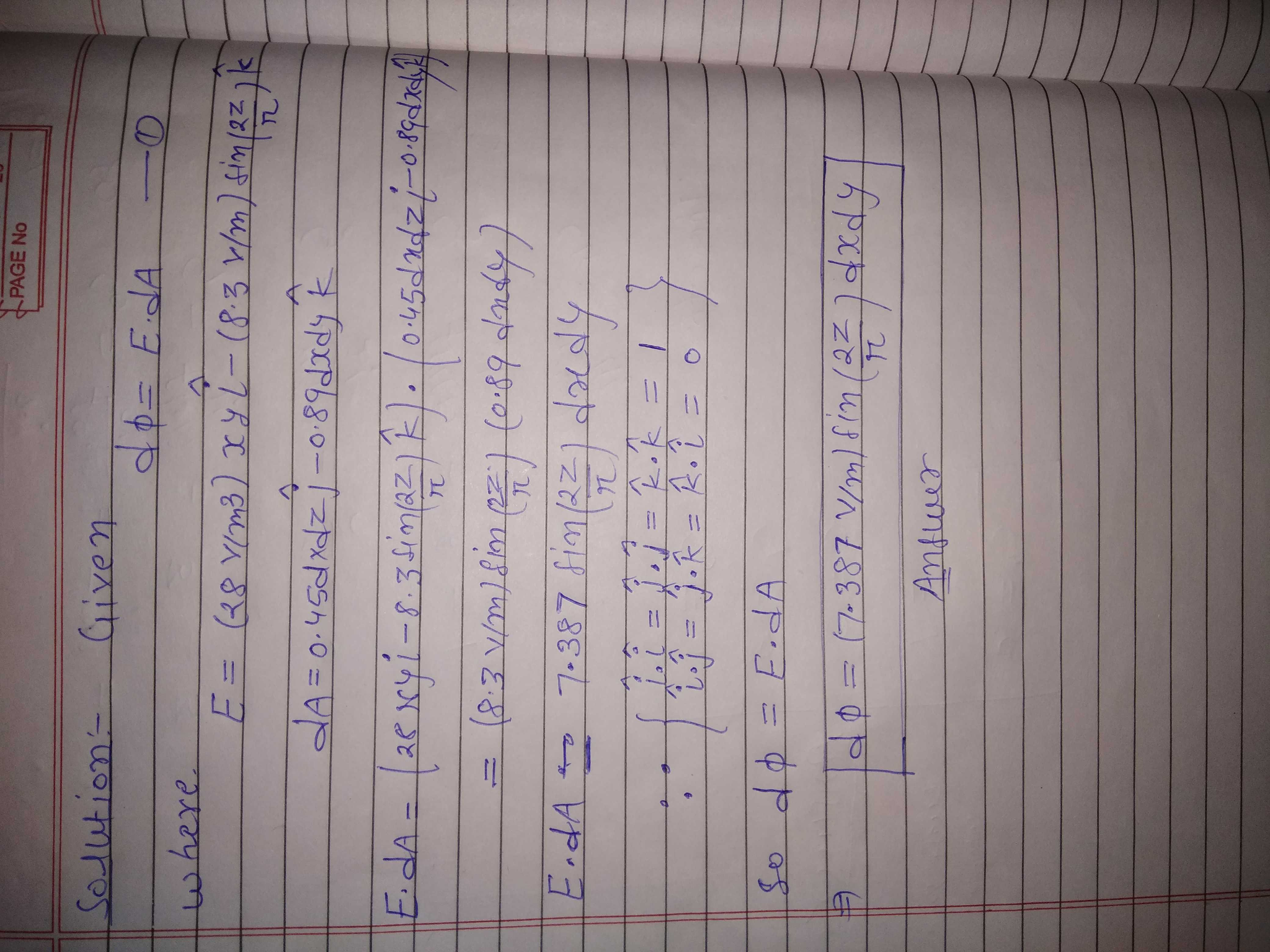##### Add Answer of: 5) dợ = E•dA, where E = (28 V/m?) xy î - (8.3 V/m) sin(2z/n) k,...
Similar Homework Help Questions
• ### 5) do = E•dA, where E = (28 V/m²) xy î - (8.3 V/m) sin(2z/n) k,...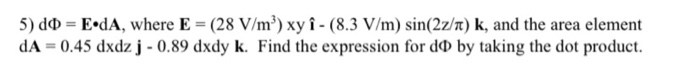5) do = E•dA, where E = (28 V/m²) xy î - (8.3 V/m) sin(2z/n) k, and the area element dA = 0.45 dxdz j - 0.89 dxdy k. Find the expression for do by taking the dot product.

• ### 2i (a) Find where M(v) - ( b) Find M() for v 2z? + 2-1-5. -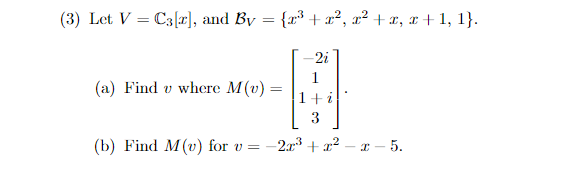2i (a) Find where M(v) - ( b) Find M() for v 2z? + 2-1-5. - 2i (a) Find where M(v) - ( b) Find M() for v 2z? + 2-1-5. -

• ### Question 8 () (- )dA, where R is the region given by 0SS1 and 0Sv (b) 2r'ydA, where R is the region given by 0 s r S 1 and s; 4. Evaluate the double integrals using polar coordinates: aRdA, wh...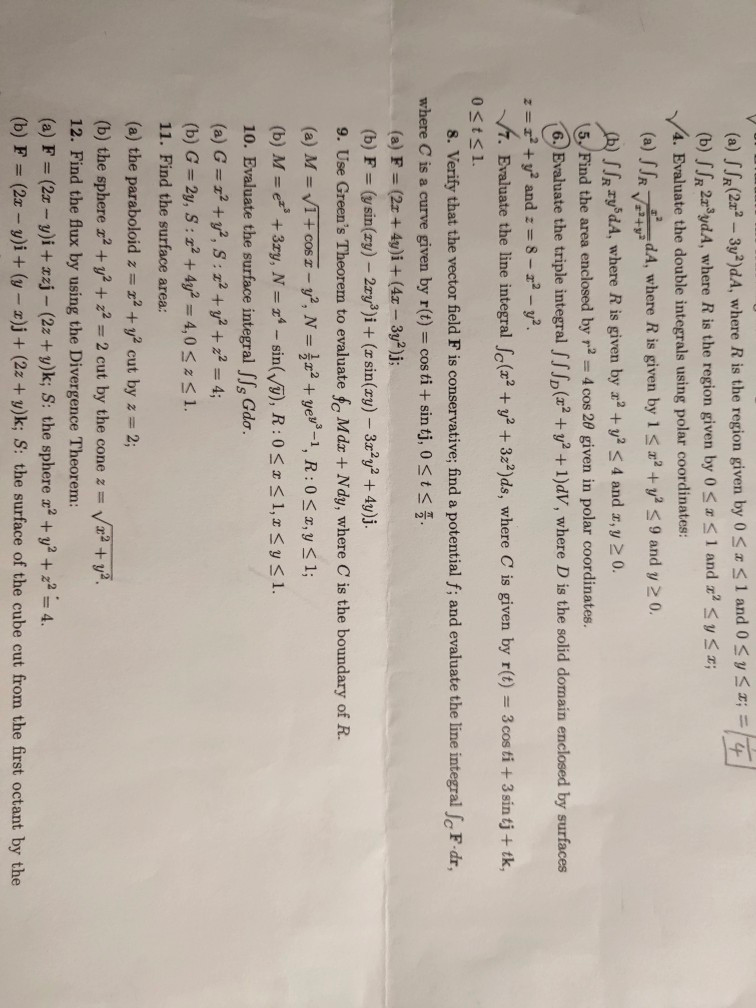Question 8 () (- )dA, where R is the region given by 0SS1 and 0Sv (b) 2r'ydA, where R is the region given by 0 s r S 1 and s; 4. Evaluate the double integrals using polar coordinates: aRdA, where R is given by 1 s + v s 9 and y 20. JJR ry'dA, where R is given by +v2 S 4 and z, y 20. 5/Find the area enclosed by r2-4 cos 2θ given in polar coordinates....

• ### At a certain instant, a particle-like object is acted on by a force F = (3.0 N) i - (1.0 N) j + (8.0 N) k while the object's velocity is v = - (2.0 m/s) i + (4.0 m/s) k

At a certain instant, a particle-like object is acted on by a force F = (3.0 N) i - (1.0 N) j + (8.0 N) k while the object's velocity is v = - (2.0 m/s) i + (4.0 m/s) k. What is the instantaneous rate at which the force does work on the object? (b) At some other time, the velocity consists of only a j component. If the force is unchanged, and the instantaneous power is -12 W,...

• ### 5 points) A spring is suspended vertically from a fixed support. The spring has spring constant k=28 N m−1k=28 N m−1. An...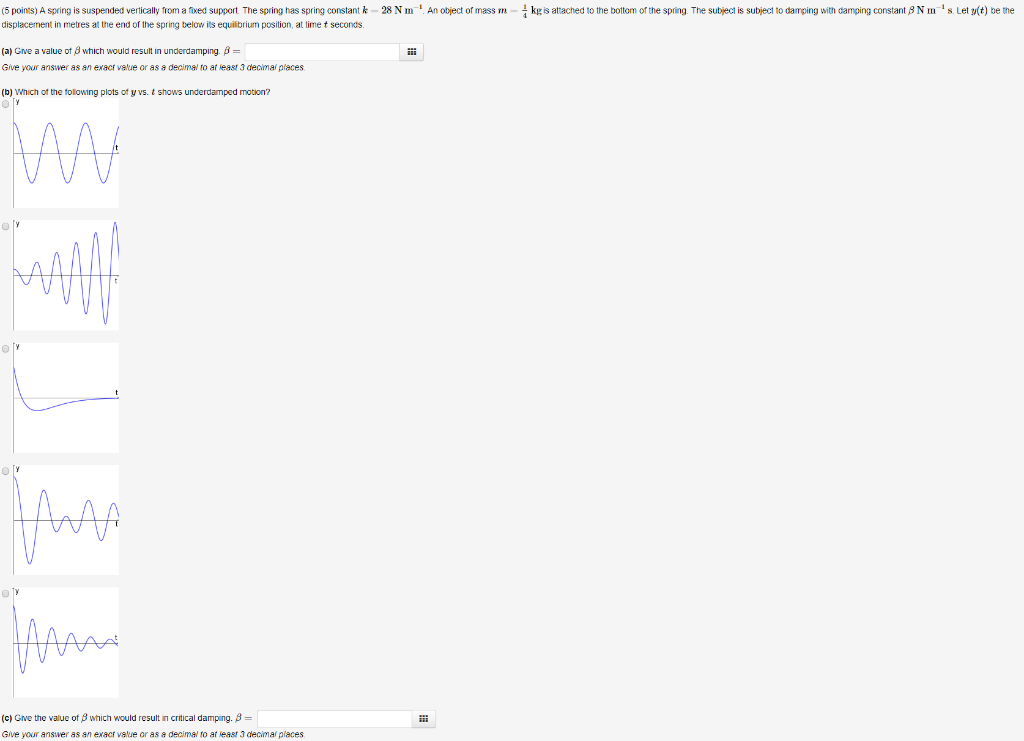5 points) A spring is suspended vertically from a fixed support. The spring has spring constant k=28 N m−1k=28 N m−1. An object of mass m=14 kgm=14 kg is attached to the bottom of the spring. The subject is subject to damping with damping constant β N m−1 sβ N m−1 s. Let y(t)y(t) be the displacement in metres at the end of the spring below its equilibrium position, at time tt seconds. (5 poins) A spring is suspended vertically...

• ### (5 points) A spring is suspended vertically from a fixed support. The spring has spring constant k=28 N m−1k=28 N m−1. A...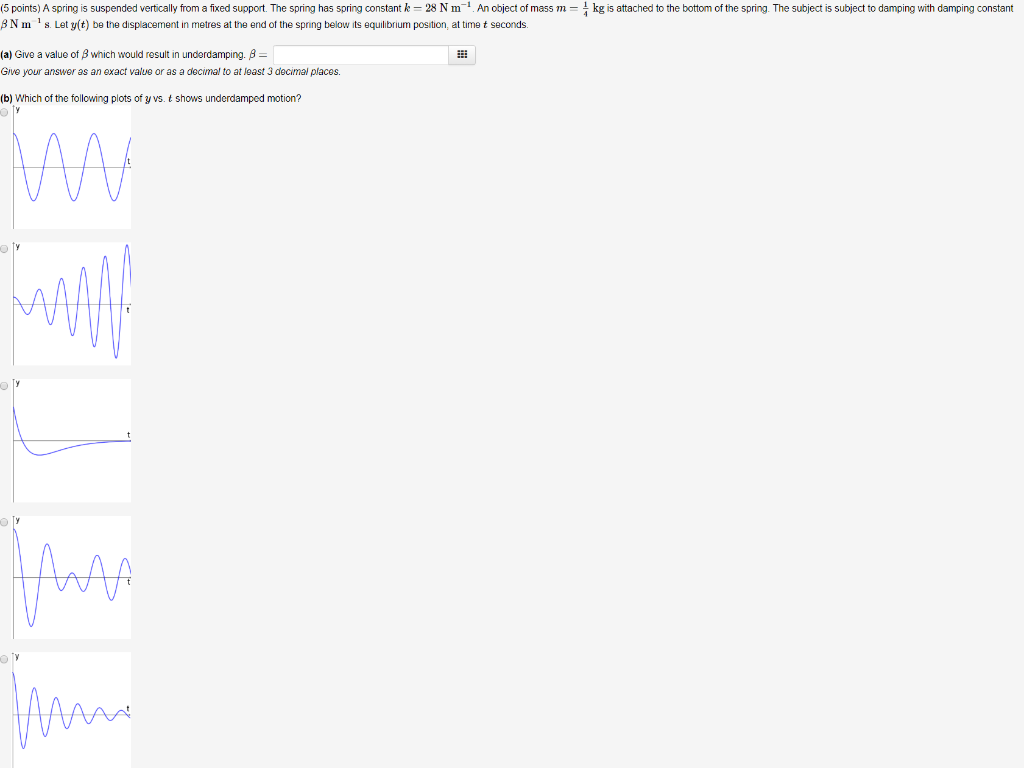(5 points) A spring is suspended vertically from a fixed support. The spring has spring constant k=28 N m−1k=28 N m−1. An object of mass m=14 kgm=14 kg is attached to the bottom of the spring. The subject is subject to damping with damping constant β N m−1 sβ N m−1 s. Let y(t)y(t) be the displacement in metres at the end of the spring below its equilibrium position, at time tt seconds. (a) Give a value of ββ which...

• ### 3. Now, consider the MOSFET DA on Figure P1.3. Transistors Q5 and Q6 are n-channel MOSFETs with threshold voltages V,-V,-V-2 V and transconductance parameters k,-k,- k- 100 m/y2 Let lc, In, and I...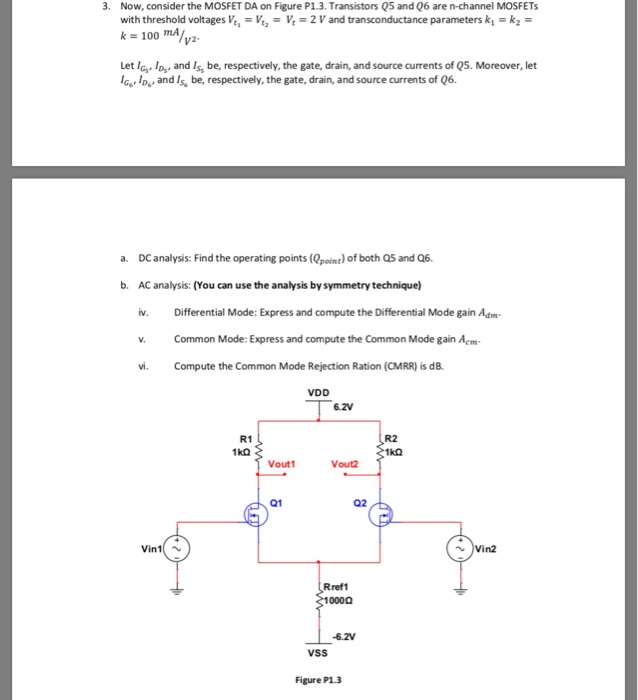3. Now, consider the MOSFET DA on Figure P1.3. Transistors Q5 and Q6 are n-channel MOSFETs with threshold voltages V,-V,-V-2 V and transconductance parameters k,-k,- k- 100 m/y2 Let lc, In, and Is, be, respectively, the gate, drain, and source currents of Q5. Moreover, let aIn, and Is, be, respectively, the gate, drain, and source currents of Q6. DC analysis: Find the operating points (Qpoine)of both Q5 and Q6. AC analysis: (You can use the analysis by symmetry technique) vDifferential...

• ### may you please do all, and explain it. will upvote it Given a hollow spherical conducting...may you please do all, and explain it. will upvote it Given a hollow spherical conducting shell (in electrostatic equilibrium) has an inner radius R1 and an outer radius R2 with a total charge -2Q distributed uniformly on its surfaces The inside of the hollow spherical conducting shell is filled with nonconducting gel with a total charge of -3Q distributed as p por (where po is a constant) through out the volume 1) Use Gauss's Law to find an expression...

• ### Hi need help for these Questions: a. Given f = yi + xzk and g = xyz2, determine (∇ x f ) . ∇g at the point (1,0,3) b. Point A lies on the curve r(t) = 2 cos t i + 2 sin t j + t k for the range 0 ≤ t...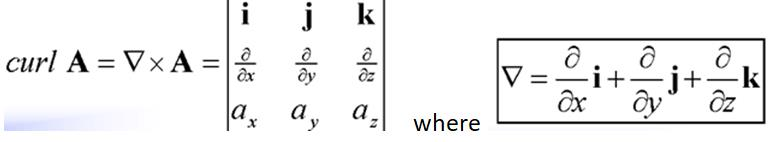Hi need help for these Questions: a. Given f = yi + xzk and g = xyz2, determine (∇ x f ) . ∇g at the point (1,0,3) b. Point A lies on the curve r(t) = 2 cos t i + 2 sin t j + t k for the range 0 ≤ t ≤ 2π . At point A, the tangent vector is T = - 21/2i + 21/2j + k. Determine the co-ordinates of point A and...

• ### #6 Letter C, can you please explain how you got the answer. and to check the answer key says its 1/144 Math 5C- Review 3 -Spring 19 1.) Evaluate. a) (c.) Jp z cos() dA, Dis bounded by y 0, y- 2, and...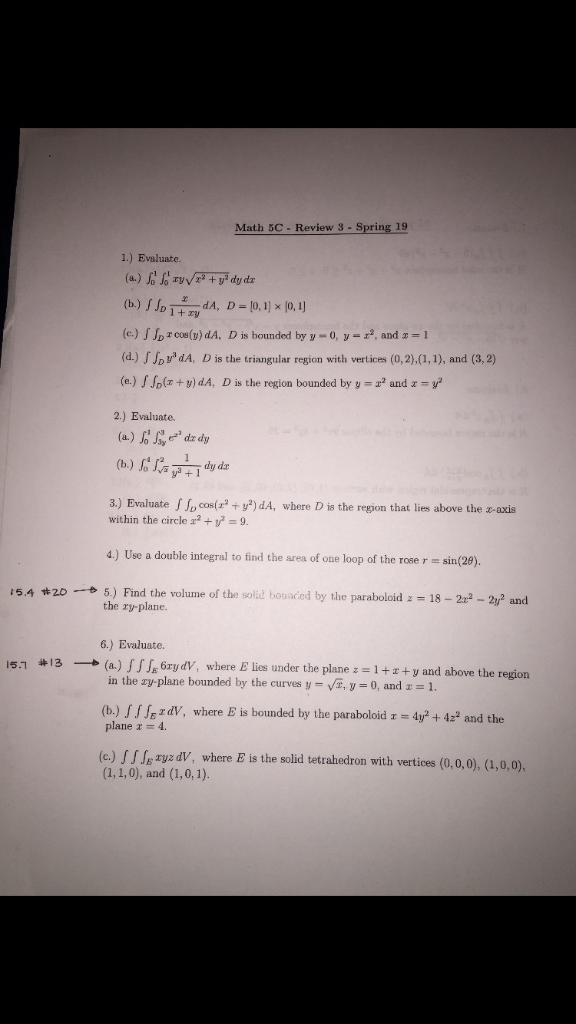#6 Letter C, can you please explain how you got the answer. and to check the answer key says its 1/144 Math 5C- Review 3 -Spring 19 1.) Evaluate. a) (c.) Jp z cos() dA, Dis bounded by y 0, y- 2, and 1 (d.) vd dA, D is the triangular region with vertices (0,2),(1,1), and (3,2) (a.) olr+v) dA, D is the region bounded by y and z 2.) Evaluate 3.) Evaluate J p cos(r +y)dA, where D is...

Free Homework App